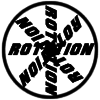#### You may also like### 8 Methods for Three by One

This problem in geometry has been solved in no less than EIGHT ways by a pair of students. How would you solve it? How many of their solutions can you follow? How are they the same or different? Which do you like best?### Rots and Refs

Follow hints using a little coordinate geometry, plane geometry and trig to see how matrices are used to work on transformations of the plane.### Reflect Again

Follow hints to investigate the matrix which gives a reflection of the plane in the line y=tanx. Show that the combination of two reflections in intersecting lines is a rotation.

# Square Pair

### Why do this problem?

This problem builds students' understanding of matrix transformations in two dimensions and encourages exploration which will increase confidence at working with vectors and matrices. Insight gained from geometrical approachs leads to a better understanding of matrix algebra.

### Possible approach

Students could explore this problem by choosing a variety of different matrices and plotting the points which $(0,0), (0,1), (1,0)$ and $(1,1)$ are transformed to. Once they have built up a picture of how the square $S$ is transformed, they could be challenged to find matrices which would transform $S$ into a particular type of quadrilateral, and then to justify why some types of quadrilateral cannot be made by transforming $S$. Students may start by justifying these conjectures using their geometrical insights, but they should be encouraged to support this using appropriate matrix algebra.

The second part of the problem asks students to investigate transformations of a second square; this could be done in the same way, first by trying some numerical examples by choosing suitable matrices, then generalising from what they find and supporting their generalisations algebraically.

### Key questions

What can you say about the image of the points on a line after transformation by a matrix?
What can you say about the image of a pair of parallel lines after transformation by a matrix?

### Possible extension

Transformations for 10 offers a variety of challenging questions about the effects of matrices in two and three dimensions, with an emphasis on thinking geometrically.

### Possible support

Begin with lots of examples of transforming the points $(0,0), (0,1), (1,0), (1,1)$ by multiplying by different matrices. Plot the resulting four points each time, and share ideas about what is common to all the images.